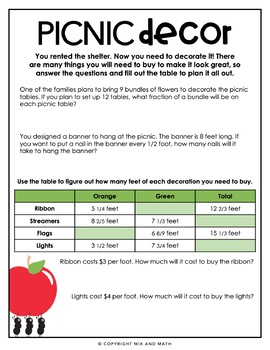# Operations with Fractions Project5th - 6th
Subjects
Standards
Resource Type
Formats Included
• PDF
•Google Apps™
Pages
20+The Teacher-Author indicated this resource includes assets from Google Workspace (e.g. docs, slides, etc.).

#### Also included in

1. Make math meaningful with these no prep real-world fraction projects and classroom transformations! In these projects, students are given real-world application for all operations with fractions. If you so choose, there are also instructions included to easily transform your classroom to bring these
\$13.00
\$16.00
Save \$3.00
2. Make math meaningful with these no prep real-world fraction projects and classroom transformations! In these projects, students are given real-world application for a variety of 5th grade math concepts. If you so choose, there are also instructions included to easily transform your classroom to brin
\$21.00
\$28.00
Save \$7.00

### Description

Make math meaningful with this no prep real-world fractions project and classroom transformation! In this project, students are planning for a big annual picnic while practicing their skills adding, subtracting, multiplying, and dividing fractions. If you so choose, there are also instructions included to easily turn your classroom into a picnic/cookout to bring this real-world project to LIFE! This project includes:

• Instructions for the project & instructions for a (optional) classroom makeover

• Same, easy to follow layout as other projects

• 6 student pages aligned to ALL 5th grade Common Core fraction standards {5.NF.1, 5.NF.2, 5.NF.3 5.NF.4, 5.NF.5, 5.NF.6, and 5.NF.7}

• A separate student pages document for digital classrooms

Your students will be so excited to plan a picnic for the day and truly experience math!

This math project comes in both PRINTABLE and DIGITAL formats.

If you enjoyed this mini-project, you will love these:

Save nearly 20% with the Fractions Math Project Bundle or save 25% with the Math Projects for 5th Grade Bundle!

-----------------------------------------------------------------------------------------------------

How to get TPT credits to use on future purchases:

• Go to your My Purchases page (you may need to login). Beside each purchase, you'll see a Provide Feedback button. Simply click it and you will be taken to a page where you can give a quick rating and leave a short comment for the product. Each time you give feedback, TPT gives you feedback credits that you may use towards future purchases!

Total Pages
20+
Included
Teaching Duration
N/A
Report this Resource to TpT
Reported resources will be reviewed by our team. Report this resource to let us know if this resource violates TpT’s content guidelines.

### Standards

to see state-specific standards (only available in the US).
Add and subtract fractions with unlike denominators (including mixed numbers) by replacing given fractions with equivalent fractions in such a way as to produce an equivalent sum or difference of fractions with like denominators. For example, 2/3 + 5/4 = 8/12 + 15/12 = 23/12. (In general, 𝘢/𝘣 + 𝘤/𝘥 = (𝘢𝘥 + 𝘣𝘤)/𝘣𝘥.)
Solve word problems involving addition and subtraction of fractions referring to the same whole, including cases of unlike denominators, e.g., by using visual fraction models or equations to represent the problem. Use benchmark fractions and number sense of fractions to estimate mentally and assess the reasonableness of answers. For example, recognize an incorrect result 2/5 + 1/2 = 3/7, by observing that 3/7 < 1/2.
Interpret a fraction as division of the numerator by the denominator (𝘢/𝘣 = 𝘢 ÷ 𝘣). Solve word problems involving division of whole numbers leading to answers in the form of fractions or mixed numbers, e.g., by using visual fraction models or equations to represent the problem. For example, interpret 3/4 as the result of dividing 3 by 4, noting that 3/4 multiplied by 4 equals 3, and that when 3 wholes are shared equally among 4 people each person has a share of size 3/4. If 9 people want to share a 50-pound sack of rice equally by weight, how many pounds of rice should each person get? Between what two whole numbers does your answer lie?
Apply and extend previous understandings of multiplication to multiply a fraction or whole number by a fraction.
Interpret multiplication as scaling (resizing), by: